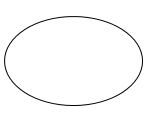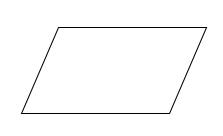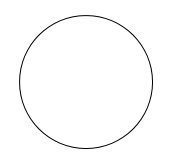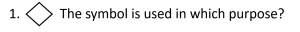# Fifth Chapter Lesson-4: Algorithm, Flowchart and Pseudo code.## At the end of this lesson-

1. You will be able to explain algorithm and flowchart.

2. You will be able to describe the conditions for writing algorithm.

3. You will be able to describe the advantages of writing algorithm and flowchart.

4. You will be able to describe the rules of creating flowchart.

5. You will be able to describe the use of symbols used in flowchart.

## Go for Bangla Version

### Algorithm:

An algorithm is a sequence of finite number of logical steps to solve a particular problem or algorithm is an ordered set of unambiguous logical steps that produces a result and terminates in a finite time. Notice the following algorithm-

An algorithm for determining average of two numbers:

Step-1: Start

Step-2: Input two numbers in variable a & b

Step-3: avg = (a+b)/2

Step-4: Print the value of avg

Step-5: Stop

The word “algorithm” relates to the name of the mathematician Al-khowarizmi, which means a procedure or a technique. Software Engineer commonly uses an algorithm for planning and solving the problems.

Conditions for creating algorithm:

1. The algorithm must be easily understandable.

2. Input and output should be defined precisely.

3. Each step in algorithm should be clear and unambiguous.

4. Each step in algorithm should have logical order.

5. Algorithm should have finite number of steps to solve a problem.

6. Algorithm should be most effective among many different ways to solve a problem.

7. An algorithm shouldn’t have computer code. Instead, the algorithm should be written in such a way that, it can be used in similar programming languages.

1. It is a step-wise representation of a solution to a given problem, which makes it easy to understand.

2. An algorithm uses a definite procedure.

3. It is not dependent on any programming language, so it is easy to understand for anyone even without programming knowledge.

4. Every step in an algorithm has its own logical sequence so it is easy to debug.

5. Assists in program change and expansion.

### Flowchart:

Flowchart uses different symbols to design a solution to a problem. In other words, Flowchart is a diagrammatic /graphical representation of an algorithm. Notice the following Flowchart-

Flowchart for determining average of two numbers:The first design of flowchart goes back to 1945 which was designed by John Von Neumann.

Flowcharts are very helpful in writing program and explaining program to others. By looking at a Flowchart one can understand the operations and sequence of operations performed in a system. Flowchart is often considered as a blueprint of a design used for solving a specific problem.

Rules of drawing flowchart:

1. Each flowchart must have only one start and one stop object.

2. The flow of control must always enter an object from the top.

3. The flow of control must always leave an object from the bottom.

4. Arrow symbol is used to show the flow of the control.

5. The conventional symbol should be used to draw a flowchart.

6. No matters if symbols become different size but shape should be exact.

7. Additional connection lines and symbols cannot be used.

8. No programming language can be used in drawing flowchart.

1. Flowchart is an excellent way of communicating the logic of a program.

2. Easy and efficient to analyze problem using flowchart.

3. During program development cycle, the flowchart plays the role of a blueprint, which makes program development process easier.

4. The flowchart makes program or system maintenance easier.

5. It is easy to convert the flowchart into any programming language code.

Types of flowchart:

System Flowchart: System Flowchart help understand how complex systems work. In system flowchart, input data, process data, store data, print result etc are shown.

Program Flowchart: Program Flowchart is a graphic representation of an algorithm, often used in the design phase of programming to work out the logical flow of a program. It demonstrates how a program works within a system. This flowchart is also used in detecting and correcting errors in a program.

To draw a Program flowchart following standard symbols are used:

 Symbol Symbol Name UseseOval Used to represent start and end of flowchart.Parallelogram Used for input and output operation.Rectangle Processing: Used for arithmetic operations and data-manipulationsDiamond Decision making. Used to represent the operation in which there are two/three alternatives, true and false etcCircle Used to join different flow line.Arrows Used to indicate the flow of logic by connecting symbols.

Basic structure of  Algorithm and flowchart:

Simple Sequence: In this structure, the instructions of a program are executed in order or sequentially.

Selection: This structure is used when instructions are executed based on the condition is true or false.

Loop: This structure is used for executing instructions repeatedly until condition is false.

Jump: In this structure, control of flow moves to a particular point based on the condition is true or false.

### Pseudo Code:

To be pseudo is to be fake. In other words, something that is pseudo is pretending that to be something it’s not. Given this, the term pseudo code makes sense – it isn’t code.

Pseudo code is an informal way of programming description that does not require any strict programming language syntax or underlying technology considerations. Notice the following Pseudo Code-

Pseudo Code for determining average of two numbers:

Start

Input a and b

avg = (a+b)/2

Print avg

Stop

It is used for creating an outline or a rough draft of a program. Pseudo code summarizes a program’s flow, but excludes underlying details. System designers write pseudo code to ensure that programmers understand a software project’s requirements and align code accordingly.

Pseudo code is not an actual programming language. So it cannot be compiled into an executable program. It uses short terms or simple English language syntaxes to write code for programs before it is actually converted into a specific programming language. The purpose of using pseudo code is an efficient key principle of an algorithm. It is used in planning an algorithm with sketching out the structure of the program before the actual coding takes place.

1. Pseudo code is understood by the programmers of all types.

2. It enables the programmer to concentrate only on the algorithm part of the code development.

## Lesson Evaluation-

Knowledge Based Questions:

a) What is algorithm?

a) What is flowchart?

a) What is pseudo code?

Comprehension Based Questions:

b) Write down the importance of algorithm in program coding.

b) “Algorithm is the precondition of coding” –explain.

b) Explain the symbols used in flowchart.

b) “Flowchart is the pictorial form of algorithm” -explain.

b) “It is convenient to solve a problem using flowchart than algorithm”-explain.

Creative Questions:

Mahin and Rakib are two friends. Mahin solved a problem using a number of simbols and Rakib solved the same problem through a series of descriptions. Eventually they wrote the program following their solution in the ‘C’ programming language.

c) Which level of programming language is mentioned in the stem? Explain.

d) Which of Mahin and Rakib’s problem solving process mentioned in the stem is more convenient? Analyze.

Multiple Choice Questions:a) Decision making       b) Processing

c) Data input                 d) Data output

2. How many types of flowcharts?

a) 2      b) 4

c) 6      d) 8i. Input

ii. Output

iii. Processing

Which one is correct?

a) i & ii       b) i & iii

c) ii & iii     d) i, ii & iii

Written by,

1.Shifana says: